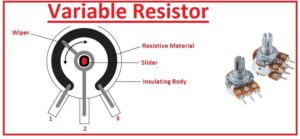Hello, readers welcome to the new post. Here we will learn How To Use Variable Resistors in Proteus. Resistance is the very basic part of any circuit that can create a hindrance in the flow of current in the circuitry. each material has a certain value of resistance and resistance or ohmmeter is used for finding the value of resistance offered by the circuitry.

The formula of resistance is R=ΡL/α. Resistance is a term used in the electrical system to explain the opposition that occurs in the flow of current. In this post, we will make a circuit and see the practical understanding of the variable resistance used in the circuit and learn its proteus simulation.

## Introduction to Variable Resistor

• The variable resistor is a category of resistor that has the ability to change its resistance measuring capacity.
• It is an electromechanical device that consists of a slider having resistance components to vary the resistance.
• If it is linked in such a way that there is a larger resistance is given then less value of current passes and in case of a small value of resistance high current passes.
• Through varying, the resistance of circuitry can also be used as a volts control device of any circuit.
• It is called a potentiometer when working as a voltage or potential divider.
• When working as a variable resistor called a rheostat.
• There is a certain type of variable resistance that operates electronically way named a digital potentiometer.

## Variable Resistor Features

• Its main features are listed here
• The physical dimensions of this resistance is larger than other resistances commonly used
• It consists of three terminals first one is a moveable pinout and the other 2 are not moveable
• It comes with a changeable port.
• It is used in small-level signal circuits.

## How To Use Variable Resistor in Proteus

• To learn the understanding of the variable resistor in proteus we will make a circuit and use the variable resistance in the proteus practically.
• First of all open the proteus on your PC then move to the component library and choose the component of projects that are listed here.
• Battery
• Resistance
• Voltmeter
• Switch
• Variable Resistance
• All components can be seen here• Make the circuit according to shown diagram and link all elements in a proper way as required.• When you make the circuit run the simulation and see the results at the output voltmeter.
• Then vary the value of variable resistance by pressing on the point of the resistor a result you will see the variation in the value of output voltages at the voltmeter.
• Can be easily seen that with the change in the resistance value of volts varying.

## Types of Variable Resistors

• Potentiometer
• Rheostat
• Digital potentiometer
• Trimmer
• A thermistor

## HOW VARIABLE RESISTORS WORK

• The variable resistor also called a potentiometer is a resistor that varies resistance according to circuit need. It is a passive and 3-terminal component that can vary resistance through the third terminal lies between two terminals so the restriction of current flow goes up and down. Variable resistors are used to adjust the value of current or voltage as the resistance of variable resistors can adjust to a specific value.
• The basic working of a variable resistor is that resistance between two endpoints can be varied through moving wiper contact with the resistive component. The wiper contact is attached to 3rd terminal and resistance between the wiper contact and any end of the point is found by the position of the wiper contact. The resistive in this module is created with material having high resistivity like carbon or metals.  The resistive element is normally helix or spiral-shaped and the wiper contact is a small metallic strip.
• The resistance of variable resistors is measured in ohms. The resistance of a variable resistor varies through the rotation of a shaft of the variable resistor. When the shaft is rotated the wiper contact moves about the resistive element and resistance between wiper contact and other endpoints of variations.## Variable Resistors vs Potentiometer

Feature Variable Resistor Potentiometer
terminals 2 3
Symbol Triangle with 2 terminals Triangle with 3 terminals
Function Resistance control in the circuit  voltage in a circuit
Applications Volume controls, dimmer switches, audio amplifiers Audio amplifiers,
Advantages Easy use  low cost a versatile device that can be used to adjust voltage or resistance
Disadvantages only be used to vary  the resistance  complex structure, costly

That is all about the How To Use Variable Resistor in Proteus if you have any further queries ask in the comments. Thanks for reading have a good day see you in the next post.